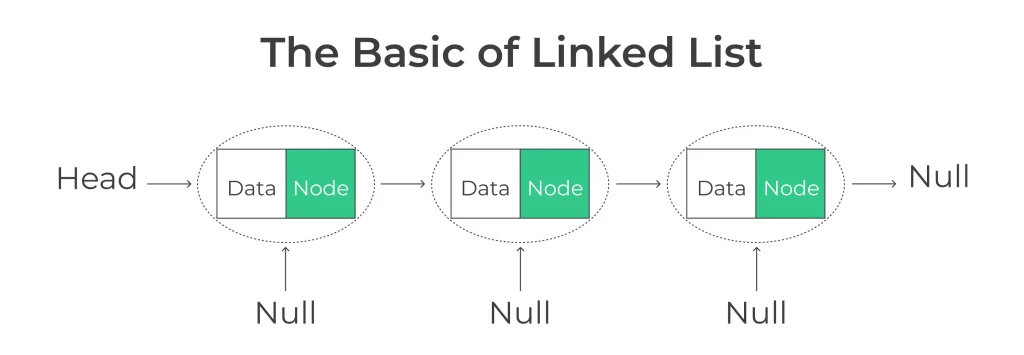## Introduction to Linked List in Python

A linked list in Python is a data structure used for storing a collection of elements, where each element, known as a “node,” contains both data and a reference to the next node in the sequence.

In this page, we will dive deep into understanding linked lists in Python, exploring their types, operations, and practical applications.## What is a Linked List in Python?

A linked list is a linear data structure where elements, known as nodes, are connected together using pointers or references. Each node contains two components: data and a reference (or link) to the next node in the sequence.

• Linked lists are versatile and can be used to implement various data structures like stacks, queues, and symbol tables.

### Types of Linked List in Python

In a singly linked list, each node points to the next node in the sequence. It is the simplest form of a linked list and is often used when we need to traverse the list in one direction.

A doubly linked list extends the concept of a singly linked list by adding an additional reference to the previous node. This bidirectional connection allows for more versatile operations but consumes additional memory.

In a circular linked list, the last node points back to the first node, forming a closed loop. This structure is advantageous in scenarios where we need continuous access to elements.### Creating a Linked List in Python

```class Node:
def __init__(self, data):
self.data = data
self.next = None

def __init__(self):
```

## Mastering Linked List Operations in Python

• Insertion Operation
• Deletion Operation

### Insertion Operation

• Insertion at the Beginning : To insert a node at the beginning of the linked list, we modify the head’ reference.
```def insert_at_beginning(self, data):
new_node = Node(data)
```
• Insertion at the End : To insert a node at the end, we traverse the list to find the last node:
```def insert_at_end(self, data):
new_node = Node(data)
return
while current.next:
current = current.next
current.next = new_node
```

### Deletion Operations

• Deleting a Node by Value : To delete a node by its value, we find the node and update the references:
```def delete_by_value(self, value):
if current and current.data == value:
current = None
return
prev = None
while current and current.data != value:
prev = current
current = current.next
if current is None:
return
prev.next = current.next
current = None
```

## Understanding Singly vs. Doubly Linked Lists

DefinitionA data structure where each node points to the next node in the list.A data structure where each node points to both the next and the previous node in the list.
NodesEach node contains data and a reference (pointer) to the next node.Each node contains data, a reference to the next node, and a reference to the previous node.
Traversing ForwardEasy and efficient.Easy and efficient.
Traversing BackwardNot efficient; requires starting from the head and traversing the list.Efficient; can traverse backward easily using the previous pointers.
Insertion/Deletion at HeadEfficient; O(1) complexity.Efficient; O(1) complexity.
Insertion/Deletion at TailInefficient; O(n) complexity as it requires traversing the entire list to reach the tail.Efficient; O(1) complexity as the tail pointer is readily accessible.
Insertion/Deletion in MiddleRequires traversal to the insertion/deletion point; O(n) complexity.Requires traversal to the insertion/deletion point; O(n) complexity.
Use CasesSuitable for cases where forward traversal is the primary operation and memory efficiency is crucial.Suitable for cases where both forward and backward traversal is required, and memory efficiency is not a significant concern.

• Dynamic Sizing: Linked lists can grow or shrink dynamically, optimizing memory usage.
• Efficient Insertions/Deletions: O(1) time complexity for inserting/deleting at the beginning.
• No Wasted Memory: Memory allocation only occurs when needed.

### Related Banners

Get PrepInsta Prime & get Access to all 200+ courses offered by PrepInsta in One SubscriptionQuestion 1.

Are linked lists only used in Python?

No, linked lists are a data structure used in many programming languages, not just Python.Question 2.

How can I optimize linked list operations for large datasets?

To optimize linked list operations for large datasets, consider using data structures like skip lists or balanced trees, which offer faster search times.Question 3.

What is the time complexity of inserting a node in a linked list?

The time complexity depends on the position of insertion. In the worst case, it’s O(n) for singly linked lists and O(1) for doubly linked lists when inserting at the beginning.

## Get over 200+ course One Subscription

Courses like AI/ML, Cloud Computing, Ethical Hacking, C, C++, Java, Python, DSA (All Languages), Competitive Coding (All Languages), TCS, Infosys, Wipro, Amazon, DBMS, SQL and others